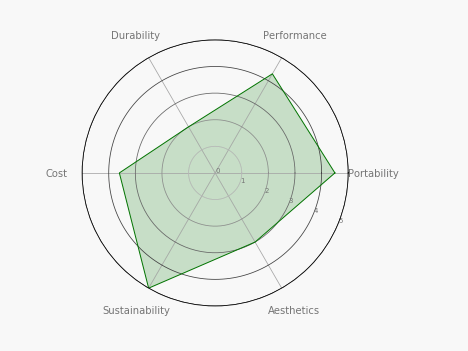Radar charts are an effective way to quickly grasp a profile of an individual item. You may have seen them before on video games or personality tests. Some other great use cases are

1. Different attributes to mark the health of a particular customer account
2. Obtaining a qualitative snapshot of competing products
3. and much more!

To create the chart above, I largely utilized the code in the Python gallery here. (This is very handy resource for all sorts of Python visualizations!) On top of this, I made a few tweaks to improve readability, aesthetics, and most notably provide automated coloring - shade the polar chart green (good), yellow (okay), red (bad) based on the scores in each attribute/criteria. Final query below

Inputs

• df: dataframe with 1 row. The first column is the name of the record, and subsequent columns are rankings for different criteria. Each criteria is a column header.
• max: maximum value to show on the r axis. In the example above, this is set to 5.
• color_by (optional parameter): gives users the option between choosing mean ranking of all criteria ('avg') and max ranking across all criteria ('max') to determine the color of the final polar plot graph. Default set to 'avg.'

Snippet

```# SQL output is imported as a pandas dataframe variable called "df"
import matplotlib.pyplot as plt
import pandas as pd
import numpy as np
from math import pi

# Function: radarchart, creates a radar-type visual from a dataframe of rankings across different attributes
# Inputs: (1) dataframe with 1 row. The first column is the name of the record, and subsequent columns are rankings for different criteria. Each criteria is a column header. (2) Maximum value to show on the r axis (3) Optional paramater that shades the shape green/yellow/red based on the ranking average ('avg') or max ranking for a single criteria 'max')
# Output: matplotlib image (polar chart) representing a radar chart

# First finding the number of variables "spokes" on the radar chart
categories=[i.capitalize() for i in list(df)[1:]]
N = len(categories)

#determining the coloring of the final shape
if (color_by=='avg'):
color_val=(df.max(numeric_only=True)).mean()
else:
color_val=(df.max(numeric_only=True)).max()
if (color_val>max*2/3):
col='green'
elif (color_val>max*1/3):
col='yellow'
else:
col='red'

values=df.loc.drop('group').values.flatten().tolist()
values += values[:1]

# Determining the angle of each spoke
angles = [n / float(N) * 2 * pi for n in range(N)]
angles += angles[:1]

# Initialize the polar plot
ax = plt.subplot(111, polar=True)
# Draw one axe per variable + add labels
plt.xticks(angles[:-1], categories, color='grey', size=10)

# Draw r axis labels
ax.set_rlabel_position(-22.5)
plt.yticks(np.linspace(0,max,6), color="grey", size=7)
plt.ylim(0,max)
a = ax.get_ygridlines()
inc=0.9/len(a)
for i in range(len(a)):
a[i].set_color((inc*(len(a)-i),inc*(len(a)-i),inc*(len(a)-i)))

# Plot data
ax.plot(angles, values, linewidth=1, linestyle='solid',color=col)

# Fill area
ax.fill(angles, values, col , alpha=0.2)

return plt

# Use Periscope to visualize a dataframe or an image by passing data to periscope.output()